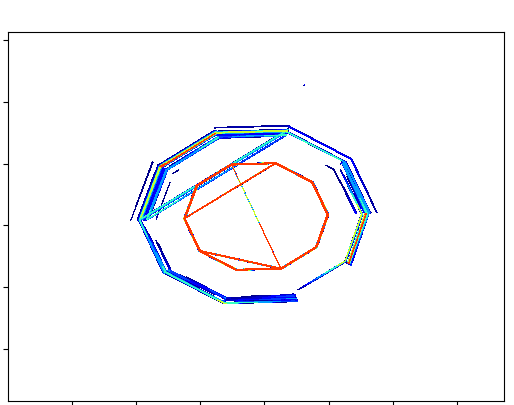# PPI data in a contour plot

I have a 2D array of data that is a result of a lidar scan through the atmosphere. Each scan through the atmosphere generates an [N, M] array of data. Where N is the laser pulse and M is the range bin. I have no issue creating a contour plot of the data in this form as it is evenly spaced.

Since the system is scanning each of the N laser pulses is at a different azimuth angle. I would like to plot the data using the latitude and longitude for each data point.
ScanLon = [n,m] array of longitudes
ScanLat = [n,m] array of latitudes
ScanData = [n,m] array of intensity values

plt.contour(ScanLon, ScanLat, ScanData)

Generates a plot, but the contours appear as lines going in a circle.I was expecting something more of a pie wedge.

I’ve tried using the triangulation as follows, but…

``````x1 = np.linspace( MinLon, MaxLon), npnts)
y1 = np.linspace(MinLat, MaxLat, npnts)
triang = tri.Triangulation(ScanLon,ScanLat)
``````

I get an error at this point “x and y must be equal-length 1D arrys”, but the rest follows from the triangulation example.

``````interpolator = tri.LinearTriInterpolator(triang,Scandata)
Xi, Yi = np.meshgrid(xi, yi)
zi = Interpolator(Xi, Yi)
``````

If you have any suggestions I would appreciate it.

Could you generate some synthetic data that fails in the same way as your real data? Being able to copy-paste-run your code will make it much easier for us to help!

In the process of writing some code to generate some simulated data I found an error in my range/bearing to lat/lon code. Once I fixed that it plotted correctly.

lat1 = Lat0 * (np.pi / 180) # system latitude in deg converted to radians
lon1 = Lon0 * (np.pi / 180) # system longitude in deg converted to radians
bearing = dataset[‘AzAngle’][None, :] * (np.pi / 180.0)
RangeVT = RangeVec[:, None] / RE
lat2 = np.arcsin(np.sin(lat1) * np.cos(RangeVT) + np.cos(lat1) * np.sin(RangeVT) * np.cos(bearing))*(180.0 / np.pi)
lon2 = Lon0 + np.arctan2(np.sin(bearing) * np.sin(RangeVT) * np.cos(lat1), np.cos(RangeVT) -
np.sin(lat1) * np.sin(lat2)) * (180.0 / np.pi)
lat2 = lat2.T
lon2 = lon2.T

Where RangeVec is an array of distanced for each range bin from the lidar system. Basically starts at zero and goes to 4 km.

Thanks for the assistance